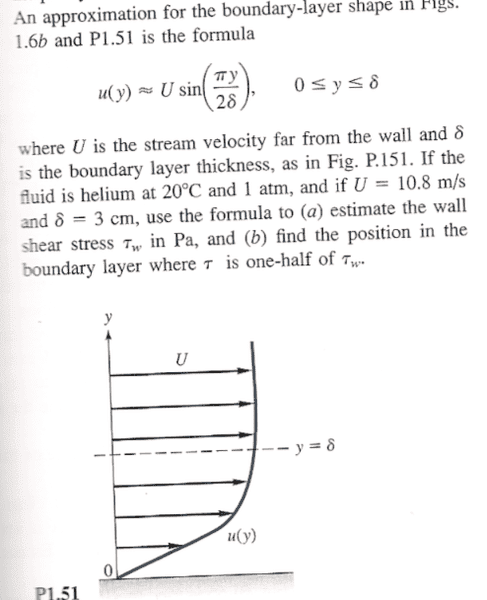# Fluid Mechanics~ Wall shear stress

## Homework StatementMy main problem here is that I do not understand what they are asking. What is the wall shear stress? Do they mean the stress at the "floor" (or whatever you want to call it)?

If so, I am assuming I use Newton's Law of Viscosity $\tau=\mu\frac{du}{dy}$ since this is 1-dimensional flow.

Would the wall shear stress $\tau_w$ be given by

$$\tau_w=\int_{y=0}^\delta \tau(y)\, dy$$

and then the problem reduces to finding an expression for $\tau(y)$

Or am I way off here? thanksI just found http://www.cfd-online.com/Wiki/Wall_shear_stress" [Broken]. So I guess my interpretation was wrong.

So, according to this definition, I should have:

$$\tau_w=\mu*\frac{d}{dy}[U\sin(\frac{\pi y}{2\delta})]|_{y=0}$$

Is that all?

Last edited by a moderator:
I just found http://www.cfd-online.com/Wiki/Wall_shear_stress" [Broken]. So I guess my interpretation was wrong.

So, according to this definition, I should have:

$$\tau_w=\mu*\frac{d}{dy}[U\sin(\frac{\pi y}{2\delta})]|_{y=0}$$

Is that all?

Not that anyone will respond to this (since no one ever looks in this forum), but I am assuming that if the above is correct, than part (b) is as simple as solving

$$\frac{1}{2}\tau_w=\mu*\frac{d}{dy}[U\sin(\frac{\pi y}{2\delta})]$$

for y.

Sound good? GoodSo I have:

$$\frac{\tau_w}{2}=\mu U\cos(\frac{\pi y}{2\delta})*\frac{\pi}{2\delta}$$

$$\Rightarrow \frac{\tau_w\delta}{\mu U\pi}=\cos(\frac{\pi y}{2\delta})$$

Last edited by a moderator:
71 views, no responses. Yesssss I am just going to keep chatting it up with myself.

Maybe I can set a new record?

Has there ever been a thread locked where there was only one speaker?

Oops! Don't answer thatI need help. (The kind PF cannot offer)

τw = μ×$\frac{d}{dy}$[Usin($\frac{\pi y}{2δ}$)]$|y=0$

so that, τw = 180$\pi$μ

and τ = μ×$\frac{d}{dy}$[Usin($\frac{\pi y}{2δ}$)] when τ = τw/2

thus, 90$\pi$μ = μ×$\frac{d}{dy}$[Usin($\frac{\pi y}{2δ}$)]

$\frac{1}{2}$ = cos($\frac{\pi y}{2δ}$)

$\frac{\pi}{3}$ = $\frac{\pi y}{2δ}$

y = 0.02 m

Chestermiller
Mentor
I just found This. So I guess my interpretation was wrong.

So, according to this definition, I should have:

$$\tau_w=\mu*[U\sin(\frac{\pi y}{2\delta})]|_{y=0}$$

Is that all?

Yes. If you actually apply the above formula, you get

τw=(μUπ)/(2δ)

Is that what you got?